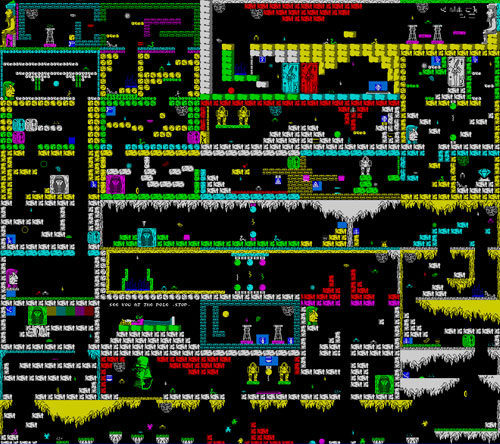# Using Photoshop to draw levels for Flash games

This is an example when things go not so good.

I was trying to draw a level for a Flash game like Tunnelball using Photoshop, and then import it into Flash.

Since it’s just an experiment, I did not draw any map but I simply downloaded one at Speccy Screenshot Maps to use it as a sample.

This is the map, its original size is 1280×1344 pixelsAnd these are the experiments. The “engine” of the “game” is the same as explained in this tutorial

First experiment

The first experiment consist in putting the map “as is”, and using it as a background.

```yspeed = 0;
xspeed = 0;
wind = 0.00;
power = 0.35;
gravity = 0.05;
upconstant = 0.75;
friction = 0.99;
_root.attachMovie("ball","ball",2,{_x:246,_y:171,_width:8,_height:8})
_root.attachMovie("map","map",1,{_x:200,_y:65})
ball.onEnterFrame = function() {
if (Key.isDown(Key.LEFT)) {
xspeed = xspeed-power;
}
if (Key.isDown(Key.RIGHT)) {
xspeed = xspeed+power;
}
if (Key.isDown(Key.UP)) {
yspeed = yspeed-power*upconstant;
}
if (Key.isDown(Key.DOWN)) {
yspeed = yspeed+power*upconstant;
}
xspeed = (xspeed+wind)*friction;
yspeed = yspeed+gravity;
map._y -=yspeed;
map._x -=xspeed;
if(map.hitTest(this._x,this._y,true)){
map._x = 200;
map._y = 65
}
};```

As you can see, the (tiny) ball in the center of the movie does not move because even if the map is “black”, flash considers the entire picture as an object, then the hitTest is always true.

Second experiment

The second experiment is the same as the first, but with the map modified with the “Trace bitmap” (Flash menu -> Modify -> Bitmap -> Trace Bitmap) and with the black areas removed.

Not only the map looksblurry, but the hitTest is not accurate…

Third experiment

It’s the same as the second, but with the traced map zoomed 4x as you can see in line 9

```yspeed = 0;
xspeed = 0;
wind = 0.00;
power = 0.35;
gravity = 0.05;
upconstant = 0.75;
friction = 0.99;
_root.attachMovie("ball", "ball", 2, {_x:246, _y:171});
_root.attachMovie("map", "map", 1, {_x:40, _y:-250, _xscale:400, _yscale:400});
ball.onEnterFrame = function() {
if (Key.isDown(Key.LEFT)) {
xspeed = xspeed-power;
}
if (Key.isDown(Key.RIGHT)) {
xspeed = xspeed+power;
}
if (Key.isDown(Key.UP)) {
yspeed = yspeed-power*upconstant;
}
if (Key.isDown(Key.DOWN)) {
yspeed = yspeed+power*upconstant;
}
xspeed = (xspeed+wind)*friction;
yspeed = yspeed+gravity;
map._y -= yspeed;
map._x -= xspeed;
if (map.hitTest(this._x, this._y, true)) {
map._x = 40;
map._y = -250;
yspeed = 0;
xspeed = 0;
}
};```

I don’t like the result… it has poor accuracy as before…

Fourth experiment

I like this experiment but I do not know how does it run on old/small computers.

I converted the orginal bitmap in a bitmapdata and performed the hit test looking at the color of the pixel at the centre of the ball (line 32).

Assuming that the “walkable” areas are all black, if the pixel has a color different than black, there is a collision

```import flash.display.BitmapData;
map = this.createEmptyMovieClip("map", 0);
map.attachBitmap(bitmap, 0);
map._x = 40;
map._y = -250;
yspeed = 0;
xspeed = 0;
wind = 0.00;
power = 0.35;
gravity = 0.05;
upconstant = 0.75;
friction = 0.99;
_root.attachMovie("ball", "ball", 2, {_x:246, _y:171});
ball.onEnterFrame = function() {
if (Key.isDown(Key.LEFT)) {
xspeed = xspeed-power;
}
if (Key.isDown(Key.RIGHT)) {
xspeed = xspeed+power;
}
if (Key.isDown(Key.UP)) {
yspeed = yspeed-power*upconstant;
}
if (Key.isDown(Key.DOWN)) {
yspeed = yspeed+power*upconstant;
}
xspeed = (xspeed+wind)*friction;
yspeed = yspeed+gravity;
map._y -= yspeed;
map._x -= xspeed;
if (bitmap.getPixel(this._x-map._x, this._y-map._y) != 0) {
map._x = 40;
map._y = -250;
yspeed = 0;
xspeed = 0;
}
};```

As I said, I am not fully satisfied of those experiment, but I would like you to tell me what do you think about.

Here you will find the source codes

214 GAME PROTOTYPES EXPLAINED WITH SOURCE CODE
// 1+2=3
// 10000000
// 2 Cars
// 2048
// Avoider
// Ballz
// Block it
// Blockage
// Bloons
// Boids
// Bombuzal
// Breakout
// Bricks
// Columns
// CubesOut
// Dots
// DROP'd
// Dudeski
// Eskiv
// Filler
// Fling
// Globe
// HookPod
// Hundreds
// InkTd
// Iromeku
// Lumines
// Magick
// MagOrMin
// Maze
// Memdot
// Nano War
// Nodes
// o:anquan
// Ononmin
// Pacco
// Phyballs
// Platform
// Poker
// Pool
// Poux
// Pudi
// qomp
// Racing
// Renju
// SameGame
// Security
// Sling
// Slingy
// Sokoban
// Splitter
// Sproing
// Stack
// Stringy
// Sudoku
// Tetris
// Threes
// Toony
// Turn
// TwinSpin
// vvvvvv
// Wordle
// Worms
// Yanga
// Zhed
// zNumbers